# OECD Gender wage pay gap data analysis 4 - Estonia is the outlier from the point of gender wage pay gap view.UnsplashRenato Pozziが撮影した写真

This post is following of the above post.

I make small data frame that contains only 2010 and 2014 observations.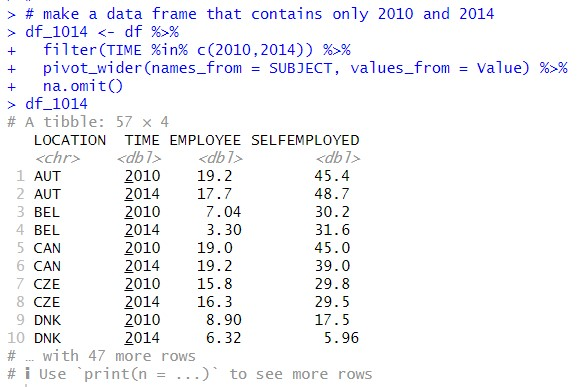First, I use filter() function to get only 2010 and 2014 data, then I use pivot_wider() function to transform data frame and I use na.omit() functuon to remove NA data.

Let's see summary statistics with summary() function.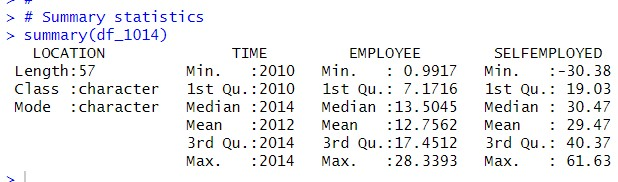I see SELFEMPLOYED has wider range than EMPLOYEE.

Let's see histogram.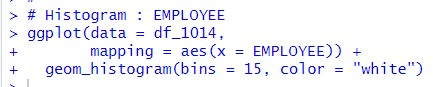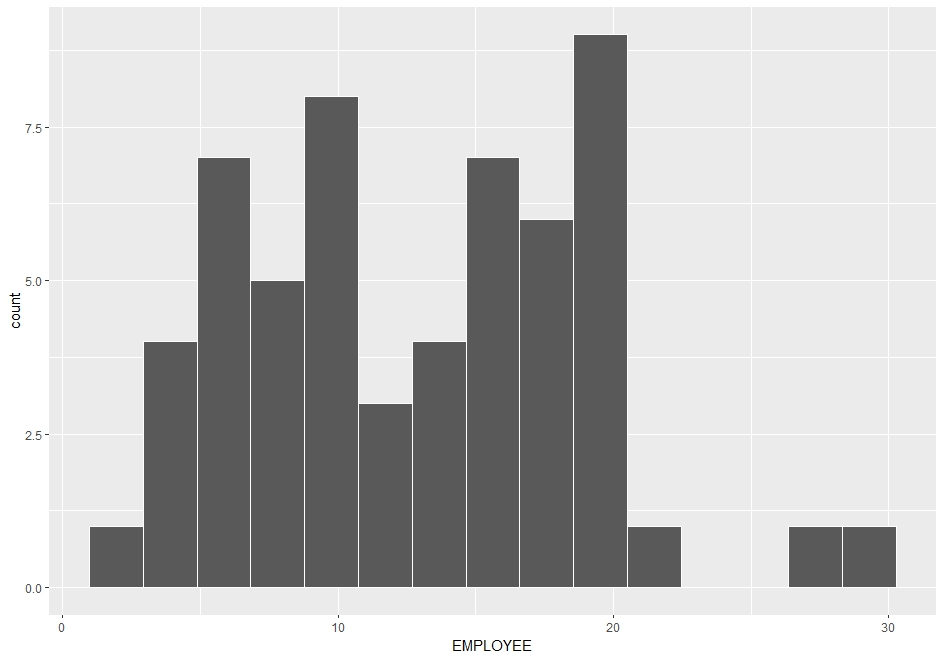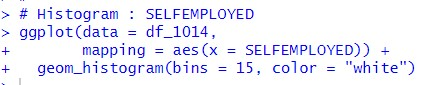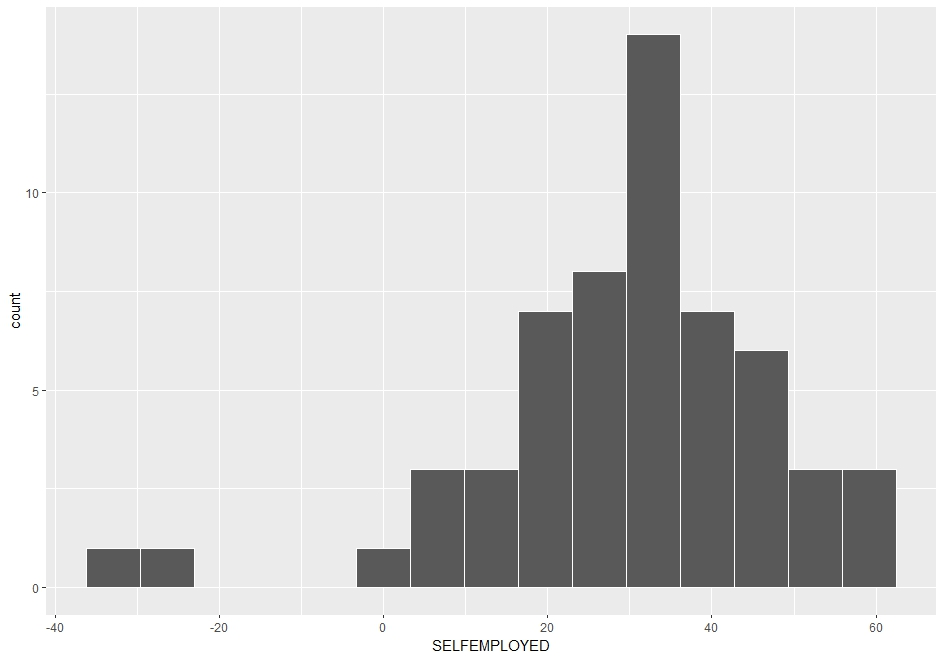I see double top for EMPLOYEE and one top for SELFEMPLOYEE.

There is outlier at larger data point in EMPLOYEE and at smaller data point in SELFEMPLOYED.

Let's see scatter plot.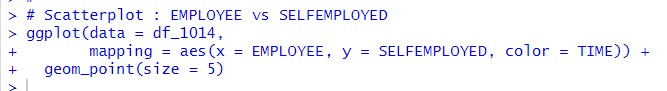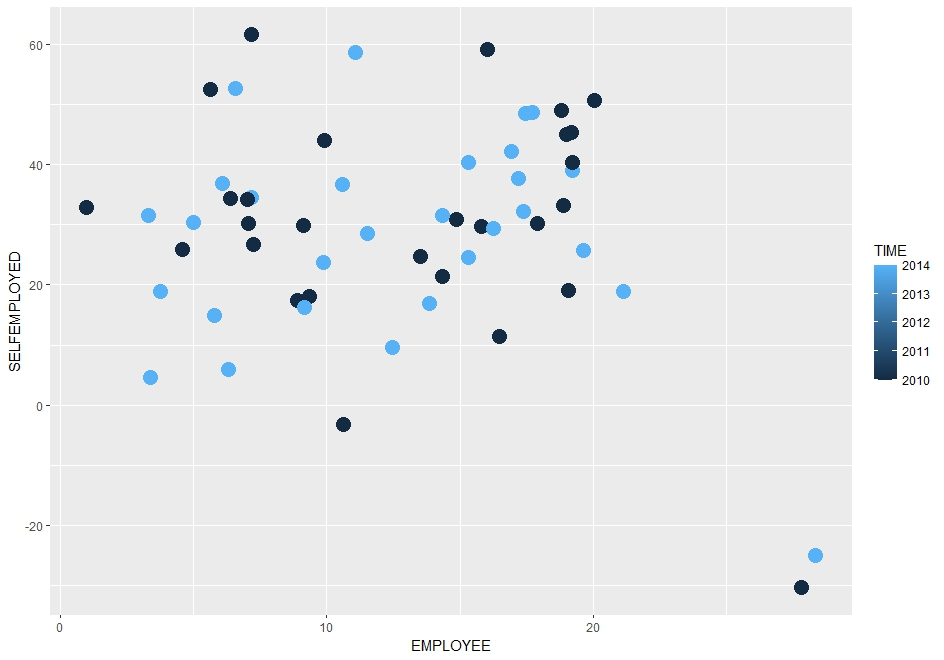At the right lower corner, there are two outliers.

Let's find which country is the outlier.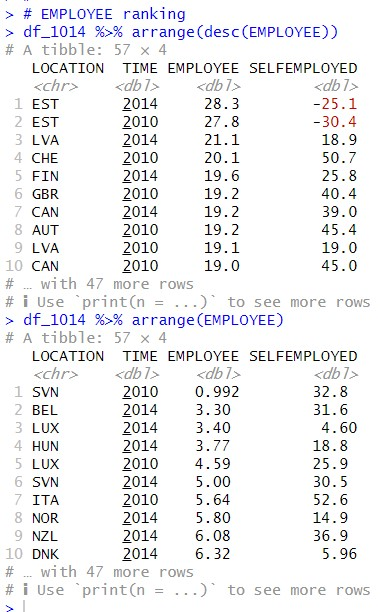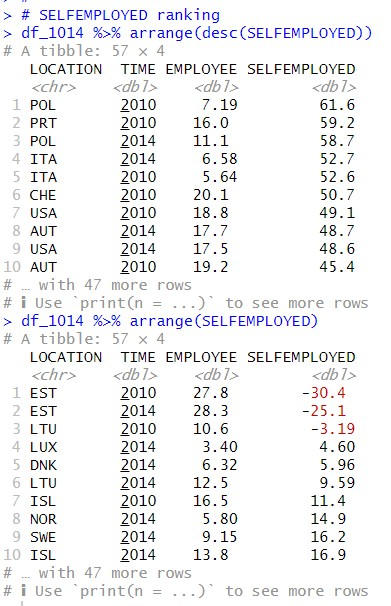I find EST is the outlier. EST is Estonia.
That's it. Thank you!

Next post is